# Working with NIFTI(-1) files in MATLAB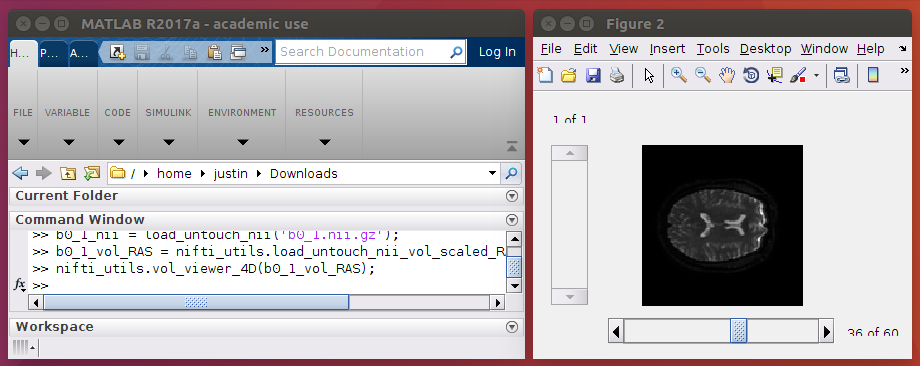This post will discuss the NIFTI-1 format and how to work with these files in MATLAB. The purpose of this article is to give a high-level introduction to “get you going”.

### What is a NIFTI file?

It’s essentially: a file containing a 3D array with an associated orientation. Technically, it’s a file containing a 348 byte header followed by image data. There’s more information here, here, and here about the specifics of what’s contained in the header. In this article, I’ll simply discuss how the orientation is stored in the header and how to use it, as well as a couple other fields which are important to properly using and manipulating the data. There are some other fields specific to certain modalities (i.e. fMRI), but they are not discussed here.

### How do I load a NIFTI file in MATLAB?

Firstly, download nifti_utils. This is actually an extension to Tools for NIfTI and ANALYZE image. nifti_utils is essentially built around the load_untouch_nii() function from this library. This function will load the NIFTI exactly as it’s stored. There’s no reorientation or data scaling or any other sort of magic under the hood. We’ll start from there and then use other more complex functions that help serve our specific purposes.

We’ll begin with two different NIFTIs, b0_1.nii.gz and b0_2.nii.gz. These data sets are simply two b0 images (non-diffusion weighted epi images) with different storage orientations.

To begin, add nifti_utils to the path and load both NIFTIs with load_untouch_nii():

%% Set environment



However, before we proceed, if you’ve already glanced at the fields stored in the NIFTI header, you’ve probably noticed there are two called scl_slope and scl_inter. NIFTIs use these fields in the following way: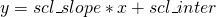Where x is the stored voxel value and y is the true value. This format is used to increase the range of values which can be stored while still using a simple integer format. For data processing purposes, the true value (usually) needs to be used. So, I recommend doing the following instead:

%% Load NIFTIs with scaled data



This function loads the image volume “untouched” (using load_untouch_nii() “under the hood”), then applies data scaling, then casts the volume to whatever type you want (in this case I use double, which is the default data type in MATLAB).

Next, view the images with:

%% View data in storage orientation
nifti_utils.vol_viewer_4D(b0_1_vol);
nifti_utils.vol_viewer_4D(b0_2_vol);



The viewers should appear like so: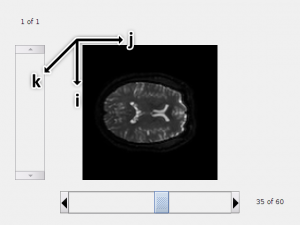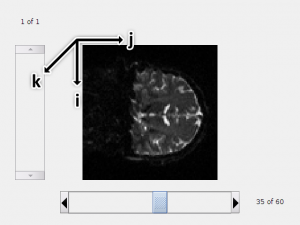The first thing to notice in the viewer is that the image storage orientations must be different. The NIFTI format deals with this issue by including an affine transform in the NIFTI header which gives the transformation from voxel coordinates (i,j,k) to some “real” space (x,y,z). Where +x “goes to the Right”, +y “goes to the Anterior”, and + z “ goes to the Superior” (this is also known as RAS orientation). This matrix is stored in two separate ways, either in something called the qform or the sform. Either, both, or neither can be stored in the NIFTI header. If the qform is set, the qform_code is greater than 0. Likewise, if the sform is set, the sform_code is greater than 0. The main distinction between the two is that the qform is parameterized as a rotation and translation whereas the sform is a full affine transformation. Hence, the qform is usually preferred. A more detailed explanation of the two is outlined here. In reality, a lot of medical imaging software don’t quite follow the format exactly and use either formats interchangeably.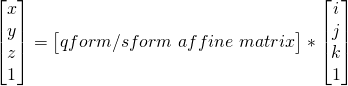### How should I use the qform/sform?

Basically, unless you have a really good or specific reason, I suggest only using them for plotting purposes. Most image processing will most naturally and easily occur with respect to storage orientation.

In order to use the qform/sform affine matrix for plotting purposes, it is generally applied in such a way that only 90 degree rotations and flips are done to the data (i.e. an approximation of the sform/qform is used instead). This is because most acquisitions aren’t perfectly on-axis, meaning if the qform/sform is applied completely, then resampling (AKA re-slicing) would need to be performed.

Using the code below:

%% Load NIFTIs with scaled data in "voxel RAS" orientation

%% View data in "voxel RAS" orientation
nifti_utils.vol_viewer_4D(b0_1_vol_RAS);
nifti_utils.vol_viewer_4D(b0_2_vol_RAS);



we can view each nifti in “voxel RAS” (AKA “RAS-ish”) form. The code first obtains the affine matrix from the qform, if it exists; if not, it uses the sform.  It then applies a threshold to the affine matrix which results in only flips and rotations. Then, it applies this transform to the 3D array. The orientations now match!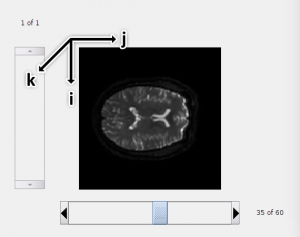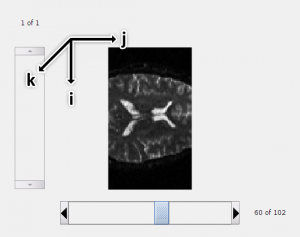As an aside, the storage orientation of the first image is LAS (the first image was flipped only in the i-th dimension). This is commonly how diffusion images are stored. The main reason to store diffusion images as LAS instead of RAS is for compatibility with a software package called FSL. I’ll discuss this in greater detail in a future article, but suffice it to say that it involves how FSL reads NIFTIs and b-vectors.

### Why are RAS oriented volumes shown as rotated axial slices in the 3D viewer?

We applied the sform/qform in such a way that (i,j,k) now maps to (x,y,z). The image viewer displays an image using something along the lines of:

imshow(data(:,:,idx1,idx2));


In MATLAB, the first index correspond to rows and the second index correspond to columns. So now we know the orientations are as shown below (where I’ve replaced (+x,+y,+z) with (R,A,S)):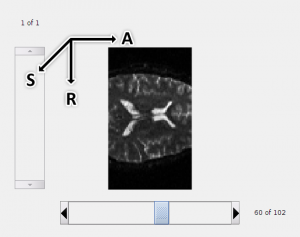This is useful to keep in mind when debugging.

### How do I save a NIFTI file in MATLAB?

Saving NIFTIs is a little more complicated than loading them. As I’ve said before, if you follow the principle of loading files in storage orientation (i.e. loading “untouched”), then saving NIFTIs usually involves simply copying over the NIFTI header information and only changing the image-related information. Most of the time you will be provided a NIFTI (i.e. through a NIFTI converter like dcm2niix) and then you will perform some image processing which usually outputs a volume the same size as the input. Because of this, the approach I’ve taken is shown below:

template_nii = load_untouch_nii(nifti_path);
template_nii.img = new_vol;
nifti_utils.save_untouch_nii_using_scaled_img_info(new_nifti_path,template_nii,'double');



This function copies everything over, except it will alter the dim field to account if the dimensions change (note that only the 4th or greater dimension should change, as a change to the first three dimensions may require recomputing the sform/qform), sets the appropriate datatype and bitpix depending on the type specified, and then sets a default scl_slope of 1 and scl_inter to 0.